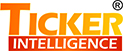## Ticker Intelligence

### Ticker IntelligenceTicker Intelligence® is the value addition section in the Market View Terminal consisting of following analytical tools as on date.

Econometric Tool:
Econometrics Tool calculates mean value and volatility of the contract.
Under Econometric Tool, Study, Econometric Tool Up, Econometric Tool and Econometric Tool Down are calculated and plotted in the chart at 30 minutes’ interval intraday.

VWAP Cumulative:
VWAP is an abbreviation for Volume Weighted Average Price. It is calculated as total value of all trades divided by total volume.
Under VWAP Cumulative study, VWAP-U, VWAP-C and VWAP-L are calculated and plotted in the chart at 30 minutes’ interval intraday.

OI Analysis:
OI stands for Open Interest. Open interest is an outstanding position of Futures and Options contracts.
Under OI Analysis study, change in open interest for futures and options contracts are calculated and plotted in the chart during the day.

Ratios:
Under Ratios, following three ratios are calculated,
Sharpe Ratio: It is calculated as average excess return divided by total risk
Sortino Ratio: It is calculated as average excess return divided by downside risk.
Treynor Ratio: It is calculated as average excess return divided by systematic risk.
Under Ratios study, above three ratios for equity stocks are calculated and plotted in the chart once in a month.

### Features

• AI tools for forecasting
• News summarizer
• Decision making tools based on deep learning
• Social media sensitivity and option analytics
• Features to aid forecasting
• Real-time analytics using millions of data sources
• Analytics based on machine learning
• Chat bots
• NLP/ NLU capability